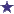# Cefazolin Dilution Pharmacy Math Question

by Thomas
(VA)Cefazolin Dilution From a Stock of 1G/5mL

Prepare 15 mL of a 50 mg/mL cefazolin dilution from a stock of 1 g/5 mL. How many milliliters of diluent and stock solution will be needed?

I am having such a hard time getting these types of calculations? I just dont get this at all?? Can you show how you worked it out?

### Comments for Cefazolin Dilution Pharmacy Math Question

Average RatingRatingAnswer by: Anonymous You can find your answer this way..1g=1000mg you want all units to be the same before you start equations.Once you convert..15mL x 50mg/1mL x 5mL/1000mg= 3.75mL of your stock needed.To find dilution simply subtract the 15mL you started with from the quantity needed..15mL-3.75mL=11.25mL

 RatingUse the Equation: Density = Mass/Volume by: Anonymous Pharmacy work involves being able to create solutions or dilutions with a variety of different medications. In some cases, these are not as easy as simply pulling our jars and bottles and measuring an amount. In some cases, the stock solution may be much stronger than the necessary dilution calls for; this means that as a pharmacy technician dealing with medication you need to be able to make the necessary dilution. Start by looking at everything you are going to need. You need a 15 mL Cefazolin solution. The stock medication Cefazolin is presented in a 1g/5mL stock strength. The solution however, requires a 50mg/mL Cefazolin solution dilution. In order to complete the dilution you need to figure out how much Cefazolin you need to add to how much of the dilution solution in order to create the necessary 15mL solution.There are several different ways to answer this problem; the easiest way will be to run the calculations in terms of density, mass, and volume.The basic formula you will need is: Density = Mass/Volume Step 1: Set up the first equation. Take the following pieces of data: the final solution amount or volume 15mL and the density of the stock solution within the final solution, which is 50mg/mL. Density = 50mg/mL 50mg/mL = Mass/15mL Volume = 15mL Step 2: You want to remove the mL from each side of the equation. You do this by multiplying by 15mL on each side. This leaves you with Mass = 50mg * 15, which gives you 750mg. This means that the final amount of the stock solution in the final solution is 750mg. This will give you the 50mg/mL dilution. You still need to determine how much of the stock solution is necessary to obtain this amount. This brings you to the second equation. You will still be working with density. Step 3: Density = Mass/Volume. In this equation, you have the density 1g/5ml = 0.75g/volume. Step 4: Multiple each side so that the equation becomes Volume = Mass/Density. The 1g/5ml will convert into a decimal of 0.2. Step 5: This gives you the following equation: Volume = 0.75/0.2. This gives you a volume of 3.75mL of the stock solution is necessary along with 11.25mL of the solution being used for the dilution to create the ratio of 50mg/mL of the stock drug in the final 15mL solution.